## Machine Learning Walkthrough

Sentiment analysis is the task of classifying text documents according to the sentiment expressed by the writer (or speaker in case of a transcription). Sentiment analysis has several applications in areas such as marketing, where online comments, reviews, and messages provide a wealth of data about customers that can be leveraged towards improved brand and customer relationship management strategies.

One way to approach this problem is to use a bag of words model. In a bag of words model, we represent the document as a numerical vector, where each element of the vector counts the number or times a word (or sequence of words) appears in the document, or simply whether the word appears in the text (leading to a binary vector). This is of course a substantial simplification since it disregards the linguistic structure of the document.

This notebook relies on the following imports and settings.

In :
# Packages
import pandas as pd
import numpy as np
import matplotlib.pyplot as plt
import seaborn as sns
import warnings
warnings.filterwarnings('ignore')

In :
# Plot settings
sns.set_context('notebook') # optimises figures for notebook display
sns.set_style('ticks') # set default plot style
crayon = ['#4E79A7','#F28E2C','#E15759','#76B7B2','#59A14F',
'#EDC949','#AF7AA1','#FF9DA7','#9C755F','#BAB0AB']
sns.set_palette(crayon) # set custom color scheme
%matplotlib inline
plt.rcParams['figure.figsize'] = (9, 6)


### 1. Twitter Airline Sentiment Data¶

In this lesson we use the Twitter Arline Sentiment dataset scraped by a company called Crowdflower and made available Kaggle datasets page. To build this dataset, the data scientists at Crowdflower scraped all tweets addressed at US airlines in the month of February 2015, including metadata about the message. Human contributors then categorised each tweet according to the sentiment (positive, neutral, or negative) expressed by the author, which can be done through services like Amazon Mechanical Turk.

In :
data=pd.read_csv('Data/Tweets.csv')
data.tail()

Out:
tweet_id airline_sentiment airline_sentiment_confidence negativereason negativereason_confidence airline airline_sentiment_gold name negativereason_gold retweet_count text tweet_coord tweet_created tweet_location user_timezone
14635 569587686496825344 positive 0.3487 NaN 0.0000 American NaN KristenReenders NaN 0 @AmericanAir thank you we got on a different f... NaN 2015-02-22 12:01:01 -0800 NaN NaN
14636 569587371693355008 negative 1.0000 Customer Service Issue 1.0000 American NaN itsropes NaN 0 @AmericanAir leaving over 20 minutes Late Flig... NaN 2015-02-22 11:59:46 -0800 Texas NaN
14637 569587242672398336 neutral 1.0000 NaN NaN American NaN sanyabun NaN 0 @AmericanAir Please bring American Airlines to... NaN 2015-02-22 11:59:15 -0800 Nigeria,lagos NaN
14638 569587188687634433 negative 1.0000 Customer Service Issue 0.6659 American NaN SraJackson NaN 0 @AmericanAir you have my money, you change my ... NaN 2015-02-22 11:59:02 -0800 New Jersey Eastern Time (US & Canada)
14639 569587140490866689 neutral 0.6771 NaN 0.0000 American NaN daviddtwu NaN 0 @AmericanAir we have 8 ppl so we need 2 know h... NaN 2015-02-22 11:58:51 -0800 dallas, TX NaN

In sentiment analysis, it is common to use hierachical classifiers that first classify a document as expressing a neutral or non-neutral sentiment, and then classify the sentiment in the documents that are predicted to have polarity. Therefore, we simplify our analysis to consider only positive and negatives tweets. Furthermore, we only keep the tweets that were classified with full confidence by the human workers.

In :
data=data[data['airline_sentiment']!='neutral']
data=data[data['airline_sentiment_confidence']==1.0]


The filtered dataset has 8897 observations.

In :
len(data)

Out:
8897

Problem formulation: our objective to build a classifier that achieves maximum accuracy in distiguishing positive and negative messages.

Here are examples of negative and positive tweets respectively.

In :
data.loc[4126, 'text']

Out:
'@united stuck here in IAH waiting on flight 253 to Honolulu for 7 hours due to maintenance issues. Could we have gotten a new plane!?!? Fail'
In :
data.loc[8644, 'text']

Out:
'@JetBlue had a great flight to Orlando from Hartford a few weeks ago! Was great to get out on time and arrive early!'

### 2. Text Processing¶

Text analysis requires careful processing of the raw text data to convert documents into a format that is amenable to analysis. We implement four steps:

1. Tokenization: separate the text into tokens (words) for a bag of words representation.
2. Removing uninformative punctuation.
3. Removing stopwords (non-discriminative words such as "the" and "to").
4. Stemming and lemmatization: converting words into a root form. Say, it can be more useful to consider "democracy", "democracies", "democratic", and "democratization" as the same token.

If this is your first time using the Natural Language Toolkit package, you'll have to do the following to download the corpora.

In :
import nltk


I'll illustrate the process for a single tweet. First, we convert the text into tokens.

In :
from nltk.tokenize import TweetTokenizer

tweet=data.loc[8644, 'text']
Tokenizer = TweetTokenizer()
tokenized = Tokenizer.tokenize(tweet)

print('Original:')
print(tweet)
print('\nTokenized:')
print(tokenized)

Original:
@JetBlue had a great flight to Orlando from Hartford a few weeks ago! Was great to get out on time and arrive early!

Tokenized:
['@JetBlue', 'had', 'a', 'great', 'flight', 'to', 'Orlando', 'from', 'Hartford', 'a', 'few', 'weeks', 'ago', '!', 'Was', 'great', 'to', 'get', 'out', 'on', 'time', 'and', 'arrive', 'early', '!']


Second, we remove the punctuation and convert all characters to lowercase. The exclamation mark may be informative about the sentiment, so I'll keep it as a token.

In :
import string
punctuation = list(string.punctuation)
punctuation.remove('!')
tokenized_no_punctuation=[word.lower() for word in tokenized if word not in punctuation]
print(tokenized_no_punctuation)

['@jetblue', 'had', 'a', 'great', 'flight', 'to', 'orlando', 'from', 'hartford', 'a', 'few', 'weeks', 'ago', '!', 'was', 'great', 'to', 'get', 'out', 'on', 'time', 'and', 'arrive', 'early', '!']


Third, we remove the stopwords.

In :
from nltk.corpus import stopwords
tokenized_no_stopwords=[word for word in tokenized_no_punctuation if word not in stopwords.words('english')]
print(tokenized_no_stopwords)

['@jetblue', 'great', 'flight', 'orlando', 'hartford', 'weeks', 'ago', '!', 'great', 'get', 'time', 'arrive', 'early', '!']


There are different methods for stemming and lemmatization available in the NLTK package. We pick one below.

In :
from nltk.stem.porter import PorterStemmer
tokens = [PorterStemmer().stem(word) for word in tokenized_no_stopwords]
print(tokens)

['@jetblu', 'great', 'flight', 'orlando', 'hartford', 'week', 'ago', '!', 'great', 'get', 'time', 'arriv', 'earli', '!']


We put all these steps below into a function that we can apply to the tweets to create a data column containing the tokens.

In :
%%time

# this cell may take over a minute to run

from num2words import num2words

def process_text(text):
tokenized = Tokenizer.tokenize(text)
punctuation = list(string.punctuation)
punctuation.remove('!')
tokenized_no_punctuation=[word.lower() for word in tokenized if word not in punctuation]
tokenized_no_stopwords=[word for word in tokenized_no_punctuation if word not in stopwords.words('english')]
tokens = [PorterStemmer().stem(word) for word in tokenized_no_stopwords if word != '️']
for i in range(len(tokens)):
try:
tokens[i]=num2words(tokens[i])
except:
pass

# Applies the process_text function separately to each element of the column 'text'
data['tokens']=data['text'].apply(process_text)

Wall time: 42.4 s


Let's have a look at the results.

In :
data[['text','tokens']].head(10)

Out:
text tokens
3 @VirginAmerica it's really aggressive to blast... [@virginamerica, realli, aggress, blast, obnox...
4 @VirginAmerica and it's a really big bad thing... [@virginamerica, realli, big, bad, thing]
5 @VirginAmerica seriously would pay $30 a fligh... [@virginamerica, serious, would, pay, thirty, ... 9 @VirginAmerica it was amazing, and arrived an ... [@virginamerica, amaz, arriv, hour, earli, good] 11 @VirginAmerica I &lt;3 pretty graphics. so muc... [@virginamerica, <3, pretti, graphic, much, be... 12 @VirginAmerica This is such a great deal! Alre... [@virginamerica, great, deal, !, alreadi, thin... 14 @VirginAmerica Thanks! [@virginamerica, thank, !] 16 @VirginAmerica So excited for my first cross c... [@virginamerica, excit, first, cross, countri,... 17 @VirginAmerica I flew from NYC to SFO last we... [@virginamerica, flew, nyc, sfo, last, week, f... 18 I ❤️ flying @VirginAmerica. ☺️👍 [❤, fli, @virginamerica, ☺, 👍] Finally, we encode the response variable numerically. In : data['positive']=(data['airline_sentiment']=='positive').astype(int) data.head()  Out: tweet_id airline_sentiment airline_sentiment_confidence negativereason negativereason_confidence airline airline_sentiment_gold name negativereason_gold retweet_count text tweet_coord tweet_created tweet_location user_timezone tokens positive 3 570301031407624196 negative 1.0 Bad Flight 0.7033 Virgin America NaN jnardino NaN 0 @VirginAmerica it's really aggressive to blast... NaN 2015-02-24 11:15:36 -0800 NaN Pacific Time (US & Canada) [@virginamerica, realli, aggress, blast, obnox... 0 4 570300817074462722 negative 1.0 Can't Tell 1.0000 Virgin America NaN jnardino NaN 0 @VirginAmerica and it's a really big bad thing... NaN 2015-02-24 11:14:45 -0800 NaN Pacific Time (US & Canada) [@virginamerica, realli, big, bad, thing] 0 5 570300767074181121 negative 1.0 Can't Tell 0.6842 Virgin America NaN jnardino NaN 0 @VirginAmerica seriously would pay$30 a fligh... NaN 2015-02-24 11:14:33 -0800 NaN Pacific Time (US & Canada) [@virginamerica, serious, would, pay, thirty, ... 0
9 570295459631263746 positive 1.0 NaN NaN Virgin America NaN YupitsTate NaN 0 @VirginAmerica it was amazing, and arrived an ... NaN 2015-02-24 10:53:27 -0800 Los Angeles Eastern Time (US & Canada) [@virginamerica, amaz, arriv, hour, earli, good] 1
11 570289724453216256 positive 1.0 NaN NaN Virgin America NaN HyperCamiLax NaN 0 @VirginAmerica I &lt;3 pretty graphics. so muc... NaN 2015-02-24 10:30:40 -0800 NYC America/New_York [@virginamerica, <3, pretti, graphic, much, be... 1

Let's save our work so far. We only keep the columns which we are going to use later.

In :
data=data[['airline','tokens','airline_sentiment','positive']]
data.to_hdf('Data/tweets_processed.h5', 'data')


### 3. Train-Test Split¶

We need to split the data into training and test sets before exploratory data analysis.

In :
from sklearn.model_selection import train_test_split

# Randomly split indexes
index_train, index_test  = train_test_split(np.array(data.index), train_size=0.7,
random_state=1, stratify=data['positive'])

# Write training and test sets
train = data.loc[index_train,:].copy()
test =  data.loc[index_test,:].copy()


Stratification means the training and test sets have the same proportion of negative and positive messages.

### 4. Exploratory Data Analysis¶

4.1 Proportion of positive versus negative messages

We start with some exploratory data analysis for the training data. We find that overall 83% of the tweets are negative and 17% are positive.

In :
train['airline_sentiment'].value_counts()

Out:
negative    5167
positive    1060
Name: airline_sentiment, dtype: int64
In :
train['airline_sentiment'].value_counts(normalize=True).round(3)

Out:
negative    0.83
positive    0.17
Name: airline_sentiment, dtype: float64

We can use cross tabulation to break down the numbers by airline.

In :
table = pd.crosstab(train['airline_sentiment'], train['airline'])
table

Out:
airline American Delta Southwest US Airways United Virgin America
airline_sentiment
negative 1133 494 620 1351 1483 86
positive 160 235 261 126 204 74

American, US Airways, and United in particular had a high proportion of negative messages that month.

In :
table = pd.crosstab(train['airline_sentiment'], train['airline'], normalize='columns').round(3)
table

Out:
airline American Delta Southwest US Airways United Virgin America
airline_sentiment
negative 0.876 0.678 0.704 0.915 0.879 0.538
positive 0.124 0.322 0.296 0.085 0.121 0.462

In graphical format:

In :
fig, ax = plt.subplots()
(table.T).plot(kind='bar', alpha=0.9, ax=ax)
ax.set_xlabel('Airline')
ax.set_ylabel('Proportion')
ax.legend_.set_title('Sentiment')
plt.tight_layout()
sns.despine()
plt.show()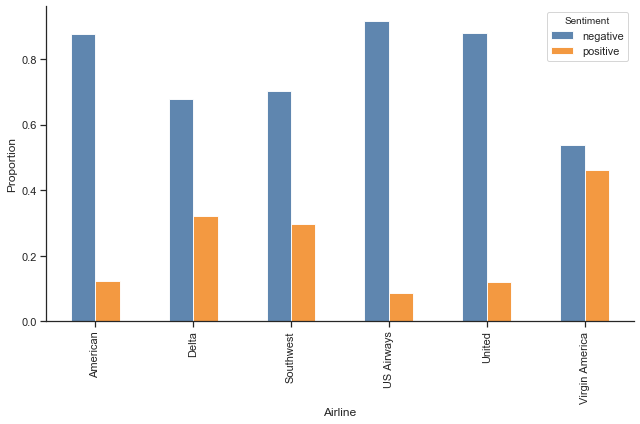4.2 Most common tokens

The NLTK FreqDist allow us to build a frequency distribution for the tokens.

In :
fdist = nltk.FreqDist()
for words in train['tokens']:
for word in words:
fdist[word] += 1

print(f'Number of unique tokens: {len(fdist)}')

Number of unique tokens: 7808


The most common tokens in the data as a whole are:

In :
fdist.most_common()[:20]

Out:
[('!', 2554),
('flight', 2330),
('@unit', 1727),
('@usairway', 1528),
('@americanair', 1391),
('@southwestair', 895),
('thank', 749),
('hour', 746),
('@jetblu', 740),
('get', 737),
('servic', 584),
('delay', 579),
('cancel', 576),
('custom', 558),
('two', 512),
('time', 510),
('help', 462),
('call', 459),
('wait', 442),
('...', 442)]

With a bit of work, we can turn this into a plot.

In :
fig, ax = plt.subplots()

y = pd.Series(dict(fdist.most_common()[:20]))
y = y.sort_values(ascending=False)

y.plot()

indexes = np.arange(0, len(y)) # we will place ticks for every word
ax.set_xticks(indexes)
ax.set_xticklabels(y.index, rotation='-90')
ax.set_xlim(-1)

plt.tight_layout()

sns.despine()
plt.show()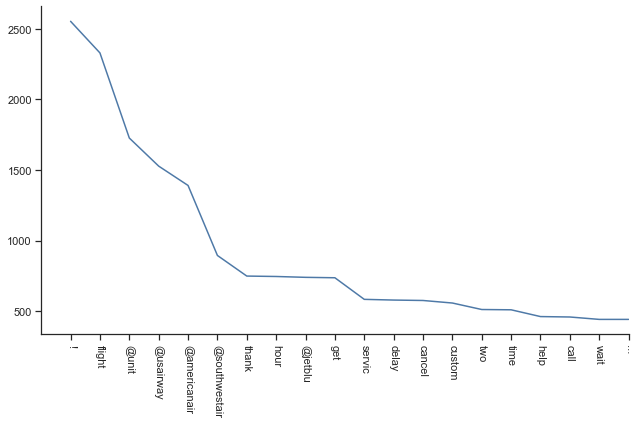4.3 Most common tokens by sentiment

Now, let's do the same for positive and negative tweets separately. Here, we compute the proportion of tweets in which the word appears and then sort the tokens accordingly in descending order.

This analysis gives us important clues for building a classifier. First, nearly half of the positive tweets contain the "thank" word, suggesting that this will be a powerful feature for classification. Not surprisingly, word such as "great", "love", "best", and "good" are among the most frequent in the positive tweets, and do not appear among the most frequent negative tweets. In the same way, "delay", "cancel", and "wait" are among the most frequent for negative tweets and are not among the most frequent among positive tweets.

Interestingly, the exclamation mark is much more common among positive tweets, confirming that it was useful to keep it.

A third group of words such as "flight" and "service" are frequent among both positive and negative tweets, and thus may not be very useful for classification.

In :
positives=len(train[train['airline_sentiment']=='positive']) # number of positive tweets in the training data

fdist_positive = nltk.FreqDist()
for words in train[train['airline_sentiment']=='positive']['tokens']:
for word in np.unique(words): # not counting repeated words this time
fdist_positive[word] += 1

common_positive = pd.Series(dict(fdist_positive))/positives
common_positive = common_positive.sort_values(ascending=False)

Out:
!                 0.592
thank             0.492
@southwestair     0.248
@jetblu           0.222
@unit             0.193
flight            0.180
@americanair      0.158
@usairway         0.123
great             0.114
servic            0.084
love              0.078
help              0.071
@virginamerica    0.070
custom            0.061
fli               0.060
good              0.060
awesom            0.057
much              0.057
best              0.056
guy               0.054
dtype: float64

We can also visualise these results as a word cloud.

In :
positive_tweets = train[train['airline_sentiment']=='positive']['tokens']

from wordcloud import WordCloud

fig, ax = plt.subplots()
wordcloud = WordCloud(background_color="white", colormap='tab10', max_words=200).generate(str(positive_tweets))
plt.imshow(wordcloud, interpolation='bilinear')
plt.axis('off')
plt.show()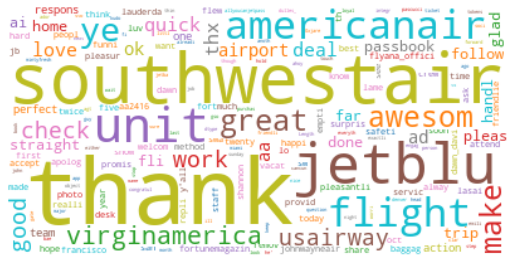In :
negatives=len(train[train['airline_sentiment']=='negative'])

fdist_negative = nltk.FreqDist()
for words in train[train['airline_sentiment']=='negative']['tokens']:
for word in np.unique(words):
fdist_negative[word] += 1

common_negative = pd.Series(dict(fdist_negative))/negatives
common_negative = common_negative.sort_values(ascending=False)

Out:
flight           0.319
@unit            0.291
@usairway        0.268
@americanair     0.236
!                0.178
hour             0.133
get              0.123
@southwestair    0.122
cancel           0.104
delay            0.101
@jetblu          0.097
servic           0.093
custom           0.092
two              0.086
time             0.082
hold             0.080
wait             0.076
call             0.076
help             0.072
...              0.069
dtype: float64
In :
negative_tweets = train[train['airline_sentiment']=='negative']['tokens']

from wordcloud import WordCloud
fig, ax = plt.subplots(figsize=(10,8))
wordcloud = WordCloud(background_color="white", colormap='tab10', max_words=200).generate(str(negative_tweets))
plt.imshow(wordcloud, interpolation='bilinear')
plt.axis('off')
plt.show()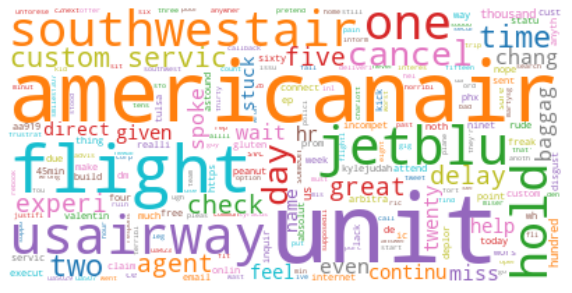4.4 Which tokens are most discriminative?

It's helpful to take our analysis one step further and formally ask which tokens are most helpful for distiguishing between positive and negative messages, when used by themselves. In this case, we can simply use Bayes' rule to compute the estimated class probabilities.

We need auxiliary code. First, we convert the frequency distribution to pandas series for convenience.

In :
vocab = pd.Series(dict(fdist))
vocab = vocab.sort_values(ascending=False)

Out:
!               2554
flight          2330
@unit           1727
@usairway       1528
@americanair    1391
dtype: int64

The next cell provides functions that perform the required calculations.

In :
from sklearn.naive_bayes import BernoulliNB
from sklearn.metrics import log_loss

# Builds a dummy variable for the presence of the token
def design_matrix(token, series):
X = series.apply(lambda tokens: (token in tokens))
X = X.astype(int)
return X.values.reshape((-1,1)) # converting to a NumPy matrix, as required

# Computes the training error from using a single token
def training_error(feature):
X_train = design_matrix(feature, train['tokens'])
nbc= BernoulliNB().fit(X_train, np.ravel(y_train))
prob = nbc.predict_proba(X_train)[:,1]
return log_loss(y_train, prob)


The results are as follows, where we rank the tokens according to the negative log-likelihood.

In :
y_train=train['positive'].values

losses=[]
for feature in vocab.index[:500]:
losses.append(training_error(feature))

ranked = pd.Series(losses, index=vocab.index[:500])
ranked = ranked.sort_values()

Out:
thank            0.352262
!                0.399451
great            0.435423
hour             0.439191
love             0.442574
awesom           0.443283
hold             0.444879
:)               0.445310
cancel           0.445428
delay            0.446736
@jetblu          0.447117
@usairway        0.447142
best             0.447376
amaz             0.447485
@southwestair    0.448180
appreci          0.448659
call             0.448795
flight           0.449184
flightl          0.449261
much             0.449794
dtype: float64

### 5. Feature Engineering¶

5.1 Bag-of-words (binary)

As mentioned in the introduction, a simple strategy is to use a bag of words model, where each predictor is a dummy binary variable indicating the presence of a token in the message.

Even though there are more than 7800 unique tokens, more than half appear only once in the training data.

In :
vocab.describe().round(0)

Out:
count    7808.0
mean        9.0
std        61.0
min         1.0
25%         1.0
50%         1.0
75%         3.0
max      2554.0
dtype: float64

Tokens with too few appearances in the training data may lead to overfitting. Hence, we set a minimum of five cases for the inclusion of a token in the model, bringing the number of features down to 1517. We're making an arbitrary choice here, but ideally we can treat this treshold as a hyperparameter.

In :
vocab = vocab[vocab >= 5]
len(vocab)

Out:
1510

Finally, we need to write a function to build the design matrix given a list of features. As a technical detail, we build it as a sparse matrix for memory efficiency. This is because the design matrix can become extremely large in this type of application (millions of entries).

In :
from scipy.sparse import csr_matrix

def design_matrix_binary(vocab, series):
X = csr_matrix((len(series),len(features))) # initialise
for i in range(len(series)):
tokens = series.iloc[i]
for j, token in enumerate(features): # scan the vocabulary
if token in tokens: # if the feature is among the tokens,
X[i, j]= 1.0
return X


We now build the final processed versions of the training and test sets.

In :
%%time

y_train = train['positive'].values
y_test = test['positive'].values

features = vocab.index
X_train = design_matrix_binary(features, train['tokens'])
X_test = design_matrix_binary(features, test['tokens'])

Wall time: 37.3 s


At this point we may again want to save the current version of the dataset if we want to use it again. Ideally we should save the feature labels too. It's easier to do this by saving the list of features in a separate file, since h5py will not accept the strings as currently encoded.

In :
import h5py
f = h5py.File('data/tweets-matrices.h5', 'w')
f.create_dataset('y_train', data=y_train)
f.create_dataset('y_test', data=y_test)
f.create_dataset('X_train', data=X_train.todense(), compression='gzip')
f.create_dataset('X_test', data=X_test.todense(), compression='gzip')
f.close()

In :
pd.DataFrame(vocab).to_csv('data/tweets_features.csv', index=False)


5.2 Bag-of-words (counts)

Another option is to a bag of words based on counts. Here, I switch to the Scikit-Learn API for convenience.

In :
from sklearn.feature_extraction.text import CountVectorizer

text_train = list(train['tokens'].apply(lambda x: ' '.join(x)))
text_test = list(test['tokens'].apply(lambda x: ' '.join(x)))
vectoriser = CountVectorizer(min_df = 5, tokenizer = lambda s: s.split(' '))
X_train_count = vectoriser.fit_transform(text_train)
X_test_count = vectoriser.transform(text_test)


5.3 TF-IDF

In the term frequency-inverse document frequency (tf-idf) approach, we scale the counts according to how common a token is. The feature associated with token that appears is nearly every document will be scaled down, while a feature associated with a token.

In :
from sklearn.feature_extraction.text import TfidfVectorizer

vectoriser = TfidfVectorizer(min_df = 5, tokenizer = lambda s: s.split(' '))
X_train_tf = vectoriser.fit_transform(text_train)
X_test_tf = vectoriser.transform(text_test)


5.4 Two-grams

In :
vectoriser = CountVectorizer(min_df = 5, ngram_range = (1,2), tokenizer = lambda s: s.split(' '))
X_train_ngram = vectoriser.fit_transform(text_train)
X_test_ngram = vectoriser.transform(text_test)


### 6. Fitting Models with Scikit-Learn¶

Scikit-learn allows us to learn and use a wide range of machine learning algorithms using a simple recipe:

1. Import the learning algorithm.
2. Specify the model and options.
3. Fit the model.
4. Use the learned model to make predictions

Here we consider the Naive Bayes classifier (NBC) as our first example. The NBC is a simple generative model commonly used in this type of application. The idea is to model to conditional distribution of the features given the classes under the assumption that the features are independent given the classes (an implausible but useful assumption for predictive purposes). We can then use Bayes' theorem to compute the class probabilities conditional on the features (given the estimated parameters).

In :
nbc = BernoulliNB()
nbc.fit(X_train, y_train)

Out:
BernoulliNB(alpha=1.0, binarize=0.0, class_prior=None, fit_prior=True)

To predict the output variable in the test data, we simply write:

In :
y_pred = nbc.predict(X_test)


### 7. Logistic Regression¶

The Scikit-Learn implementation of logistic regression uses regularisation by default, with a hyperparameter C that is the inverse of the penalty.

We can do as follows to obtain a logistic regression without regularisation.

In :
from sklearn.linear_model import LogisticRegression
logit = LogisticRegression(C=np.inf, solver='lbfgs')
logit.fit(X_train, y_train)

Out:
LogisticRegression(C=inf, class_weight=None, dual=False, fit_intercept=True,
intercept_scaling=1, max_iter=100, multi_class='warn',
n_jobs=None, penalty='l2', random_state=None, solver='lbfgs',
tol=0.0001, verbose=0, warm_start=False)

Regularisation will be probably be helpful since we have a large number of features. We use the LogisticRegressionCV class, which has in-built hyperparameter optimisation using cross-validation.

In :
from sklearn.linear_model import LogisticRegressionCV
logit_l1= LogisticRegressionCV(Cs = 50, penalty='l1', solver='liblinear', scoring='neg_log_loss')
logit_l1.fit(X_train, y_train)

Out:
LogisticRegressionCV(Cs=50, class_weight=None, cv='warn', dual=False,
fit_intercept=True, intercept_scaling=1.0, max_iter=100,
multi_class='warn', n_jobs=None, penalty='l1',
random_state=None, refit=True, scoring='neg_log_loss',
solver='liblinear', tol=0.0001, verbose=0)

With an $\ell_1$ penalty, we shrink 166 coefficients to zero.

In :
np.sum(np.abs(logit_l1.coef_) == 0.0)

Out:
1063
In :
from statlearning import plot_coefficients
plot_coefficients(logit_l1, features)
plt.show()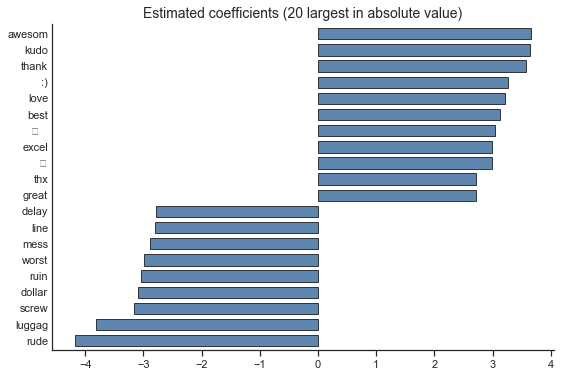In :
logit_l2= LogisticRegressionCV(Cs = 50, penalty='l2', solver='lbfgs', scoring='neg_log_loss')
logit_l2.fit(X_train, y_train)

Out:
LogisticRegressionCV(Cs=50, class_weight=None, cv='warn', dual=False,
fit_intercept=True, intercept_scaling=1.0, max_iter=100,
multi_class='warn', n_jobs=None, penalty='l2',
random_state=None, refit=True, scoring='neg_log_loss',
solver='lbfgs', tol=0.0001, verbose=0)
In :
plot_coefficients(logit_l2, features)
plt.show()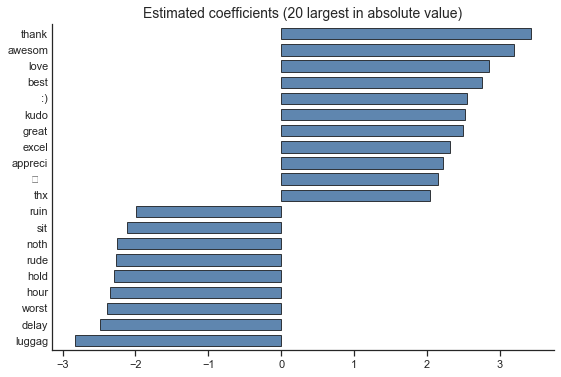### 8. Cross-Validation¶

In the previous section, we used an implementation of logistic regression with automatic selection of the regularisation parameter based on cross-validation. Let's now have a look at how we can compute a cross-validation score directly.

As an example, we will use cross-validation to select the feature engineering strategy for logistic regression. To save time, obtain model instances based on the optimal penalties (otherwise we would end doing cross-validation within cross-validation).

In :
logit_l2= LogisticRegressionCV(Cs = 50, penalty='l2', solver='lbfgs', scoring='neg_log_loss')
logit_l2.fit(X_train, y_train)
logit_l2 = LogisticRegression(C = logit_l2.C_, penalty='l2', solver='lbfgs')
logit_l2.fit(X_train, y_train)

logit_l2_count= LogisticRegressionCV(Cs = 50, penalty='l2', solver='lbfgs', scoring='neg_log_loss')
logit_l2_count.fit(X_train_count, y_train)
logit_l2_count = LogisticRegression(C = logit_l2_count.C_, penalty='l2', solver='lbfgs')
logit_l2_count.fit(X_train_count, y_train)

logit_l2_ngram= LogisticRegressionCV(Cs = 50, penalty='l2', solver='lbfgs', scoring='neg_log_loss')
logit_l2_ngram.fit(X_train_ngram, y_train)
logit_l2_ngram = LogisticRegression(C = logit_l2_ngram.C_, penalty='l2', solver='lbfgs')
logit_l2_ngram.fit(X_train_ngram, y_train)

logit_l2_tf= LogisticRegressionCV(Cs = 50, penalty='l2', solver='lbfgs', scoring='neg_log_loss')
logit_l2_tf.fit(X_train_tf, y_train)
logit_l2_tf = LogisticRegression(C = logit_l2_tf.C_, penalty='l2', solver='lbfgs')
logit_l2_tf.fit(X_train_tf, y_train)

Out:
LogisticRegression(C=11.513953993264458, class_weight=None, dual=False,
fit_intercept=True, intercept_scaling=1, max_iter=100,
multi_class='warn', n_jobs=None, penalty='l2', random_state=None,
solver='lbfgs', tol=0.0001, verbose=0, warm_start=False)

The cross_validate function from Scikit-Learn allows to computer the cross-validation error of a model.

In :
from sklearn.model_selection import cross_validate
scores = cross_validate(logit_l2, X_train, y_train, cv=5, scoring = ['accuracy','neg_log_loss'])
scores

Out:
{'fit_time': array([0.04488873, 0.0349133 , 0.03888273, 0.03788638, 0.03790116]),
'score_time': array([0.0009973 , 0.00099111, 0.00100946, 0.00099587, 0.00099564]),
'test_accuracy': array([0.95264848, 0.95505618, 0.95180723, 0.95421687, 0.94618474]),
'train_accuracy': array([0.98333668, 0.98534431, 0.9849458 , 0.9857487 , 0.98514653]),
'test_neg_log_loss': array([-0.13833589, -0.12352133, -0.12574869, -0.14041352, -0.15196999]),
'train_neg_log_loss': array([-0.06261703, -0.06336958, -0.06356099, -0.06066578, -0.06109914])}

Finally, let's build a table comparing the cross-validation results for different models.

In :
columns=['Error rate', 'Cross-Entropy']
rows=['Binary', 'Count', '2-Grams', 'TF-IDF']

results=pd.DataFrame(0.0, columns=columns, index=rows)

methods=[logit_l2, logit_l2_count, logit_l2_ngram,  logit_l2_tf]
designs=[X_train, X_train_count, X_train_ngram, X_train_tf]

for i, (method, design) in enumerate(zip(methods, designs)):

scores = cross_validate(method, design, y_train, cv=5, scoring = ['accuracy','neg_log_loss'])
results.iloc[i,0]=  1 - scores['test_accuracy'].mean()
results.iloc[i,1]=  -1*scores['test_neg_log_loss'].mean()

results.round(3)

Out:
Error rate Cross-Entropy
Binary 0.048 0.136
Count 0.050 0.139
2-Grams 0.049 0.136
TF-IDF 0.049 0.134

### 9. Hyperparamer optimisation¶

Selecting hyperparameters is one of the fundamental steps in supervised learning. In addition to using cross-validation as the model selection criterion,

As a first illustration, we implement a grid search for regularised logistic regression, which is equivalent to what LogisticRegressionCV does automatically. A grid search is not always computationally feasible. In the next section, we will look at other options for more complex models.

In :
%%time

from sklearn.model_selection import GridSearchCV

Cs = np.logspace(-10, 10, 161, base=2)
model = LogisticRegression(penalty='l2', solver='lbfgs')

space ={
'C': Cs,
}

logit_search = GridSearchCV(model, space, cv=5, scoring='neg_log_loss', n_jobs=4)
logit_search.fit(X_train, y_train)

logit_ = logit_search.best_estimator_

print('Best parameters found by grid search:', logit_search.best_params_, '\n')

Best parameters found by grid search: {'C': 2.0}

Wall time: 9.64 s


### 10. Random Forest¶

In :
from sklearn.ensemble import  RandomForestClassifier
from sklearn.model_selection import RandomizedSearchCV

In :
%%time

model = RandomForestClassifier(criterion = 'entropy',  n_estimators=100)

tuning_parameters = {
'min_samples_leaf': [1, 5, 10, 20, 50],
'max_features': np.arange(1, X_train.shape),
}

rf_search = RandomizedSearchCV(model, tuning_parameters, cv = 5, n_iter= 64, scoring='neg_log_loss', n_jobs=4)
rf_search.fit(X_train, y_train)

rf = rf_search.best_estimator_

print('Best parameters found by randomised search:', rf_search.best_params_, '\n')

Best parameters found by randomised search: {'min_samples_leaf': 1, 'max_features': 39}

Wall time: 3min 13s


11.1 XGBoost

In :
from xgboost import XGBClassifier
from skopt.space import Real, Categorical, Integer
from skopt import BayesSearchCV

In :
%%time

model = XGBClassifier(reg_lambda=0)

space = {
'learning_rate': Real(0.005, 0.15),
'n_estimators' : Integer(100, 2000),
'max_depth' : Integer(1, 4),
'subsample' : Real(0.5, 1.0),
'colsample_bynode' : Real(0.01, 1.0),
}

np.random.seed(87)
xgb_search =  BayesSearchCV(model, space, cv = 5,  n_iter=32, scoring = 'neg_log_loss', n_jobs=4)
xgb_search.fit(X_train, y_train)

xgb = xgb_search.best_estimator_

print('Best parameters found by Bayesian estimation:', xgb_search.best_params_, '\n')

Best parameters found by Bayesian estimation: {'colsample_bynode': 0.01, 'learning_rate': 0.15, 'max_depth': 2, 'n_estimators': 1490, 'subsample': 1.0}

Wall time: 4min 55s

In :
from statlearning import plot_feature_importance
plot_feature_importance(xgb, features, max_features=30)
plt.show()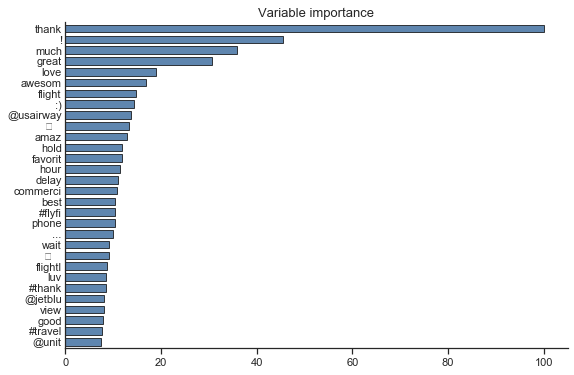11.2 LightGBM

I'm setting up the search space for LightGBM differently from XGBoost to encourage diversity between the two models.

In :
from lightgbm import LGBMClassifier

In :
%%time

model = LGBMClassifier()

space = {
'reg_alpha':  (1e-10, 1000, 'log-uniform'),
'learning_rate': Real(0.005, 0.15),
'n_estimators' : Integer(100, 2000),
'num_leaves' : Integer(2, 2**10),
'subsample' : Real(0.5, 1.0),
'colsample_bytree' : Real(0.1, 1.0),
}

np.random.seed(87)
lgb_search =  BayesSearchCV(model, space, cv = 5,  n_iter=32, scoring = 'neg_log_loss', n_jobs=4)
lgb_search.fit(X_train, y_train)

lgb = lgb_search.best_estimator_

print('Best parameters found by Bayesian optimisation:', lgb_search.best_params_, '\n')

Best parameters found by Bayesian optimisation: {'colsample_bytree': 0.22359406727251832, 'learning_rate': 0.0856106309786248, 'n_estimators': 121, 'num_leaves': 646, 'reg_alpha': 2.5132692138750777e-09, 'subsample': 0.8245674153300899}

Wall time: 4min 10s

In :
from statlearning import plot_feature_importance
plot_feature_importance(lgb, features, max_features=30)
plt.show()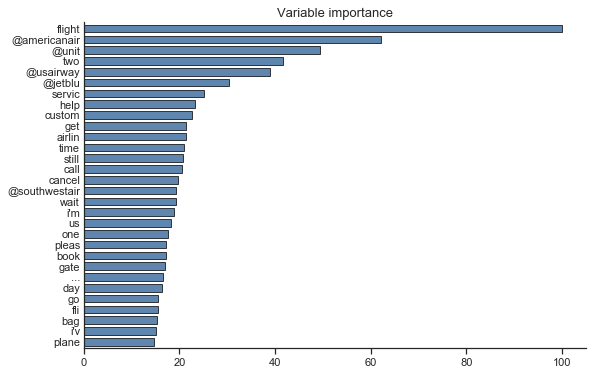### 12. Support Vector Classifier¶

In :
%%time
from sklearn.svm import LinearSVC

Cs = np.logspace(-10, 10, 161, base=2)

model = LinearSVC(loss='squared_hinge', penalty='l2', dual=False)

space ={
'C': Cs,
}

svm_search = GridSearchCV(model, space, cv=5, scoring='roc_auc', n_jobs=4)
svm_search.fit(X_train, y_train)

svm = svm_search.best_estimator_

print('Best parameters found by grid search:', svm_search.best_params_, '\n')

Best parameters found by grid search: {'C': 0.10511205190671431}

Wall time: 18.1 s


### 13. Model Stacking¶

In :
%%time
from mlxtend.classifier import StackingCVClassifier

stack = StackingCVClassifier([nbc, logit_l1, logit_l2, xgb, lgb], use_probas=True,
meta_classifier = LogisticRegression(C=np.inf, solver='lbfgs'), cv=5)
stack.fit(X_train, y_train)

Wall time: 25 s

Out:
StackingCVClassifier(classifiers=[BernoulliNB(alpha=1.0, binarize=0.0, class_prior=None, fit_prior=True), LogisticRegressionCV(Cs=50, class_weight=None, cv='warn', dual=False,
fit_intercept=True, intercept_scaling=1.0, max_iter=100,
multi_class='warn', n_jobs=None, penalty='l1',
random_s...0.0, silent=True, subsample=0.8245674153300899,
subsample_for_bin=200000, subsample_freq=0)],
cv=5, drop_last_proba=False,
meta_classifier=LogisticRegression(C=inf, class_weight=None, dual=False, fit_intercept=True,
intercept_scaling=1, max_iter=100, multi_class='warn',
n_jobs=None, penalty='l2', random_state=None, solver='lbfgs',
tol=0.0001, verbose=0, warm_start=False),
n_jobs=None, pre_dispatch='2*n_jobs', random_state=None,
shuffle=True, store_train_meta_features=False, stratify=True,
use_clones=True, use_features_in_secondary=False,
use_probas=True, verbose=0)

### 14. Model Evaluation¶

Here are the results, including common classification metrics.

In :
from sklearn.metrics import accuracy_score, confusion_matrix, roc_auc_score
from sklearn.metrics import precision_score, log_loss

columns=['Error rate', 'Sensitivity', 'Specificity', 'Precision', 'AUC', 'Cross-Entropy']
rows=['Naive Bayes', 'Logistic $\ell_1$', 'Logistic $\ell_2$', 'Random Forest',
'XGBoost', 'LightGBM', 'Linear SVC', 'Stack']

results=pd.DataFrame(0.0, columns=columns, index=rows)

methods=[nbc, logit_l1, logit_l2, rf, xgb, lgb, svm, stack]

y_prob = np.zeros((len(y_test), len(rows)))

for i, method in enumerate(methods):

y_pred = method.predict(X_test)

if method not in [svm]: # svm does not compute probabilities
y_prob[:, i] = method.predict_proba(X_test)[:,1]
results.iloc[i, 4]=  roc_auc_score(y_test, y_prob[:,i])
results.iloc[i, 5]=  log_loss(y_test, y_prob[:,i])
elif method == svm:
y_df = method.decision_function(X_test)
results.iloc[i, 4]=  roc_auc_score(y_test, y_df)

confusion  = confusion_matrix(y_test, y_pred)
results.iloc[i,0]=  1 - accuracy_score(y_test, y_pred)
results.iloc[i,1]=  confusion[1,1]/np.sum(confusion[1,:])
results.iloc[i,2]=  confusion[0,0]/np.sum(confusion[0,:])
results.iloc[i,3]=  precision_score(y_test, y_pred)

results.round(3)

Out:
Error rate Sensitivity Specificity Precision AUC Cross-Entropy
Naive Bayes 0.049 0.859 0.970 0.854 0.976 0.141
Logistic $\ell_1$ 0.050 0.791 0.983 0.905 0.974 0.138
Logistic $\ell_2$ 0.043 0.818 0.985 0.919 0.978 0.129
Random Forest 0.058 0.771 0.977 0.871 0.961 0.184
XGBoost 0.048 0.782 0.986 0.922 0.977 0.133
LightGBM 0.050 0.767 0.988 0.928 0.973 0.145
Linear SVC 0.044 0.813 0.985 0.918 0.979 0.000
Stack 0.044 0.818 0.984 0.914 0.980 0.129

### Formatting¶

The two cells below format the notebook for display online. Please omit them from your work.

In :
%%html
<style>

from IPython.core.display import HTML